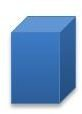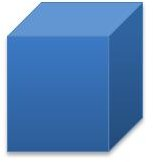# How to find the volume of a prism## Lesson Procedure: Finding the Volume of a Right Rectangular PrismLook at the right rectangular prism on the right. The measurement of the length is 2¼ inches. The measurement of the width is 1/2 inch. The measurement of the height is 1 inch.

Change the dimensions of the right rectangular prism to improper-like fractions. The dimensions are 9/4, 2/4, and 4/4 inches.

To find the volume of this right rectangular prism, fill it with ¼ inch cubes. It takes 64 – ¼ inch cubes to fill one unit cube.

The right rectangular prism can be filled with ¼ inch cubes. It takes 9 x 2 x 4 cubes to fill this right rectangular prism. It takes 72 – ¼ inch cubes to fill this right rectangular prism.

The volume of the right rectangular prism is 9/4 x 2/4 x 4/4 = 72/64 or 1 8/64 or 1 1/8 cubic inches.

## Individual or Group Work:Look at the right rectangular prisms below. Fill the prisms with unit cubes of the appropriate unit fraction edge lengths.

1. The measurement of the length is 2 inches. The measurement of the width is 1 inch. The measurement of the height is 3¾ inches. How many cubes with side lengths of ¼ would be needed to fill the prism? What is the volume of the prism? Show how you found your answers.2. The measurement of the length is 1½ inches. The measurement of the width is ½ inch. The measurement of the height is 4 inches. How many cubes with side lengths of 1/2 inch would be needed to fill the prism? What is the volume of the prism? Show how you found your answers.

Related text  How to get rid of redness from acne3. The measurement of the length is 4 1/2 inches. The measurement of the width is 1 ½ inch. The measurement of the height is 2 inches. How many cubes with side lengths of 1/2 inch would be needed to fill the prism? What is the volume of the prism? Show how you found your answers.4. The measurement of the length is 3/4 inches. The measurement of the width is ½ inch. The measurement of the height is 2 inches. How many cubes with side lengths of 1/4 inch would be needed to fill the prism? What is the volume of the prism? Show how you found your answers.5. The measurement of the length is 2½ inches. The measurement of the width is 3 ½ inch. The measurement of the height is 4 inches. How many cubes with side lengths of 1/2 inch would be needed to fill the prism? What is the volume of the prism? Show how you found your answers.